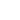Sie haben Javascript deaktiviert!
Sie haben versucht eine Funktion zu nutzen, die nur mit Javascript möglich ist. Um sämtliche Funktionalitäten unserer Internetseite zu nutzen, aktivieren Sie bitte Javascript in Ihrem Browser.Invariant Set of the Lorenz System

Attractor of the Mackey-Glass Equation

Unstable manifold of the Kuramoto-Sivashinsky equation

Petersen Graph

## Infinite Dimensional Dynamical Systems

Development of a set-oriented numerical technique which allows to compute low-dimensional invariant sets for infinite dimensional dynamical systems.

Systems of interest:

• Delay Differential Equations (DDEs): type of differential equation in which the time derivative of the unknown function depends not only on the current state but also on previous times.
Focus of interest: Analysis of long term behavior of DDEs
Applications: e.g. signal processing, disease transition, population modelsSuccessively finer coverings of a relative global attractor for the Mackey-Glass equation

• Partial Differential Equations (PDEs): type of differential equation in which the time derivative of the unknown function depends on multivariable functions and their partial derivatives.
Focus of interest: Analysis of long term behavior of PDEs
Applications: e. g. fluid dynamics, quantum mechanics, electrodynamicsBox covering of an attractor of the Kuramoto Sivashinsky equation

Contact: Adrian Ziessler

ERROR: Content Element with uid "121391" and type "media" has no rendering definition!

##### Further information:

The University for the Information Society